# 5TH ANNIVERSARY AUCTION

### Auction Details

Choose Your Layout >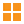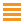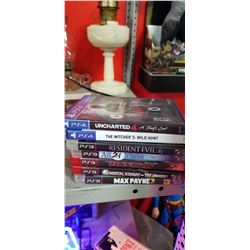181 - Shelf One
CDC Auctions
Shelf One
Bidding Has Concluded

Sold to f***r for (45.00 + 5.85) x 1 = 50.85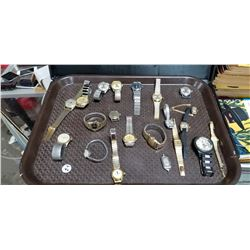182 - Shelf One
CDC Auctions
Shelf One
Bidding Has Concluded

Sold to f***r for (150.00 + 19.50) x 1 = 169.50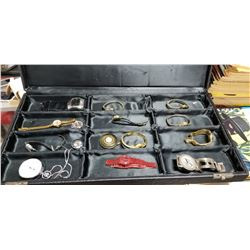183 - Shelf One
CDC Auctions
Shelf One
Bidding Has Concluded

Sold to f***r for (37.50 + 4.88) x 1 = 42.38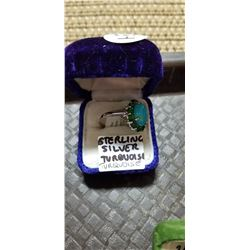185 - Shelf One
CDC Auctions
Shelf One
Bidding Has Concluded

Sold to f***r for (45.00 + 5.85) x 1 = 50.85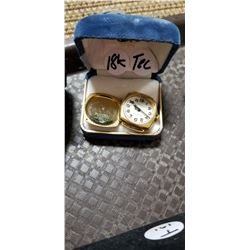187 - Shelf One
CDC Auctions
Shelf One
Bidding Has Concluded

Sold to f***r for (450.00 + 58.50) x 1 = 508.50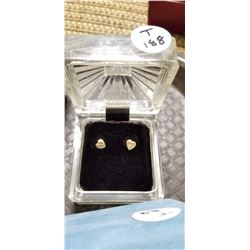188 - Shelf One
CDC Auctions
Shelf One
Bidding Has Concluded

Sold to f***r for (17.50 + 2.28) x 1 = 19.78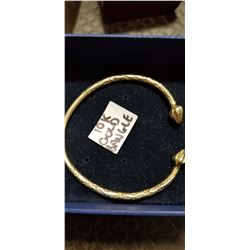189 - Shelf One
CDC Auctions
Shelf One
Bidding Has Concluded

Sold to f***r for (300.00 + 39.00) x 1 = 339.00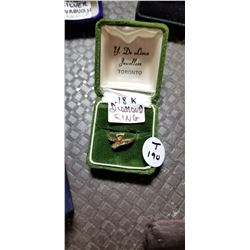190 - Shelf One
CDC Auctions
Shelf One
Bidding Has Concluded

Sold to f***r for (100.00 + 13.00) x 1 = 113.00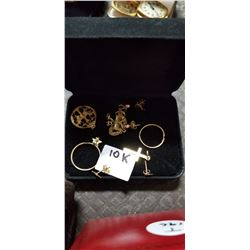191 - Shelf One
CDC Auctions
Shelf One
Bidding Has Concluded

Sold to f***r for (65.00 + 8.45) x 1 = 73.45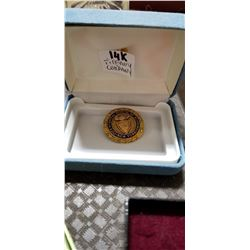192 - Shelf One
CDC Auctions
Shelf One
Bidding Has Concluded

Sold to f***r for (200.00 + 26.00) x 1 = 226.00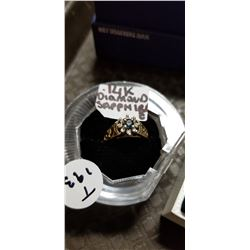193 - Shelf One
CDC Auctions
Shelf One
Bidding Has Concluded

Sold to f***r for (110.00 + 14.30) x 1 = 124.30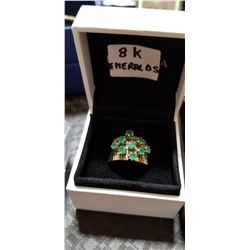194 - Shelf One
CDC Auctions
Shelf One
Bidding Has Concluded

Sold to f***r for (150.00 + 19.50) x 1 = 169.50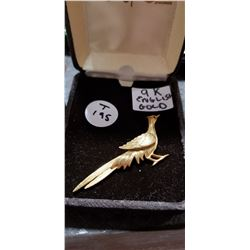195 - Shelf One
CDC Auctions
Shelf One
Bidding Has Concluded

Sold to f***r for (95.00 + 12.35) x 1 = 107.35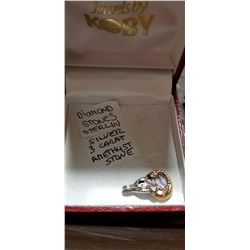196 - Shelf Two
CDC Auctions
Shelf Two
Bidding Has Concluded

Sold to f***r for (17.50 + 2.28) x 1 = 19.78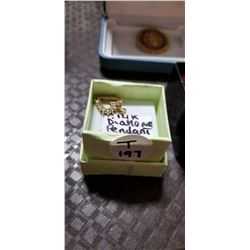197 - Shelf One
CDC Auctions
Shelf One
Bidding Has Concluded

Sold to f***r for (40.00 + 5.20) x 1 = 45.20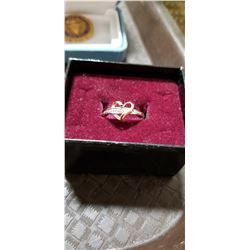198 - Shelf One
CDC Auctions
Shelf One
Bidding Has Concluded

Sold to f***r for (50.00 + 6.50) x 1 = 56.50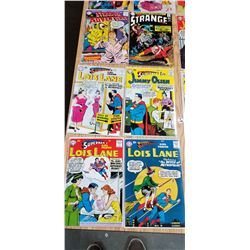199 - Shelf One
CDC Auctions
Shelf One
Bidding Has Concluded

Sold to f***r for (65.00 + 8.45) x 1 = 73.45